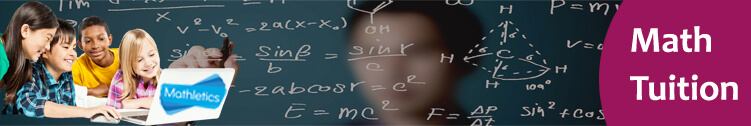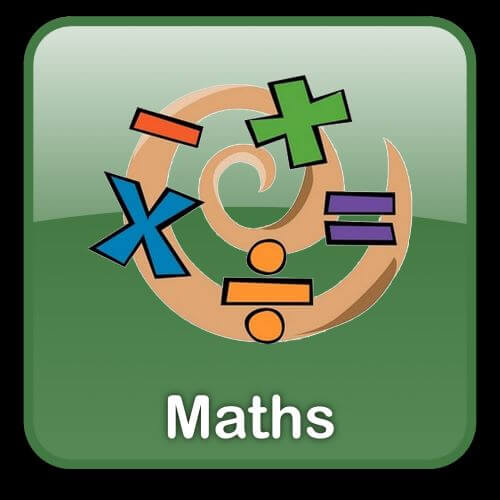## Secondary 1 Maths# Maths Tuition Centre for Secondary 1

Our Maths tuition Centre for students of secondary one aims at building interest amongst students in abstract mathematical concepts by means of various curricular activities and lesson plans. Students will be taught about basic manipulative skills such as arithmetic and algebraic manipulation and data handling.

About the course- Students studying the maths tuition will be taught many skills and techniques that will help them understand mathematics better, along with other mentioned topics below.

• Students will be taught about concepts of variables, and learn how to represent the relationship between two variables in tabular, algebraic, and graphical forms.
• Students will learn new terms related to household and personal finance such as exchange rates, installments, and interest, utility bill.
• Students will be taught how to effectively use algebra and other symbols to represent mathematical ideas.
• Students will be taught application of algebraic equations to variety of problems and on solving those problems using a systematical and logical approach.

Target students: This course is meant only for students of class secondary one who want to study mathematics both express and normal academics.

Why choose inspizone?

Inspizone has firm believes of providing high quality education with the best methods and techniques that will help students grow into elite individuals. Other than that, students gain the following benefits-

• Students are under the influence of highly educated teachers that constantly assess them, help them develop their pit holes and motivate them all the time .
• Students will be given quality study material that will be self sufficient and help students achieve excellent grades.
• Students will be surrounded in an engaging, interactive and positive atmosphere that will help them become more self confident and acquire excellent communication skills.

Schedule: Weekdays (Monday to Friday: 5.30 pm to 8.30 pm) & Saturday and Sunday: 9.30 am to 4 pm.

Fee: Please make enquiry via Whatsapp or Email or call us

Venue: 10 Anson Road, 26-08A International Plaza, Singapore 079903

##secondary 1 school mathematics (Syllabus)

### Lesson 1: Factors and Multiples

• Primes, Primes Factorisation and Index Notation
• Highest Common Factor (HCF)
• Lowest Common Multiples (LCM)
• Square Roots and Cube Roots

### Lesson 2: Real Numbers

• Idea of Negative Numbers and the Number Line
• Addition and Subtraction of integers
• Multiplication, Division and Combined Operations of Integers
• Rational Numbers
• Real Numbers and Summary of the Use of Calculators

### Lesson 3: Approximation and Estimation

• Rounding Off Numbers to Decimal Places
• Rounding Off Numbers to Significant Figures
• Estimation

### Lesson 4: Introduction to Algebra

• The Use of Letters in Algebra
• Evaluation of Algebraic Expression and Formulae
• Translation of Real – World Situations Into Algebraic Expressions

### Lesson 5: Algebraic Manipulation

• Like Terms and Unlike Terms
• Addition and Subtraction of Linear Expressions
• Simplification of Linear expressions
• Factorisation By using Common Factors

### Lesson 6: Simple Equations in One variable

• Simple Linear Equations in One Variable
• Equations Involving Brackets
• Simple Fractional Equations
• Forming Linear Equations to Solve Problems